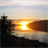PSoC™ 5, 3 & 1Level 5
Level 5

Hi

I'd like to use the ADC_SAR  and I have some question regarding using this API  :  void ADC_SetScaledGain(int16 adcGain)

According to the data sheet     adcGain = counts x 10 / Vmeasured.

This work OK , I have a prototype working based on that..

Now, assume I want to calibrate the signal chain including gain op amp and active filter and also assume that I cannot use the Delta

I can supply a known voltage  close to the reference voltage (in my case 1024 mv.)  and measure as directed

with a voltmeter  the voltage at the ADC input an get the value of adcGain.

If I wanted to make a PCB serially I would need to do this measurement on each board. ?

How automate this? Some suggestion ?

1 SolutionLevel 9Level 9

AIVa,

Instead of factory calibration against an external voltage (e.g. 1024mV) and adjusting Gain setting, consider using onboard reference Vref = 1.02V as input test voltage, and record measured value (e.g. Vmeasured = 0.99V). Then all data need to be corrected for coefficient: coeff = Vref/Vmeasured. This procedure can be run every time on startup (with some MUX), so no factory calibration required.

High-precision absolute voltage measurement can be tricky if better than 10^-3 resolution is needed. Usually, a stable external voltage source is used for calibration (like Mercury-Silver battery) on every measurement, as internal Vref drifts away. If accuracy of few mV is sufficient, then existing PSoC factory calibration of ADC should suffice.

/odissey1

3 RepliesLevel 9Level 9

AIVa,

Instead of factory calibration against an external voltage (e.g. 1024mV) and adjusting Gain setting, consider using onboard reference Vref = 1.02V as input test voltage, and record measured value (e.g. Vmeasured = 0.99V). Then all data need to be corrected for coefficient: coeff = Vref/Vmeasured. This procedure can be run every time on startup (with some MUX), so no factory calibration required.

High-precision absolute voltage measurement can be tricky if better than 10^-3 resolution is needed. Usually, a stable external voltage source is used for calibration (like Mercury-Silver battery) on every measurement, as internal Vref drifts away. If accuracy of few mV is sufficient, then existing PSoC factory calibration of ADC should suffice.

/odissey1Level 5
Level 5

Odissey1

Thank you.

You said

 record measured value (e.g. Vmeasured = 0.99V).

Can you clarify how you get those (in this case )  0.99V ?

1- Using the same SAR_ADC ?

2- Reading the counts  you get from 1.02 V ?Level 9Level 9